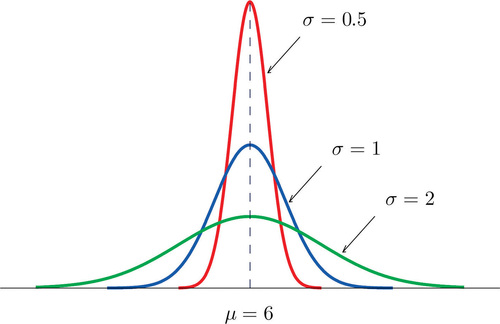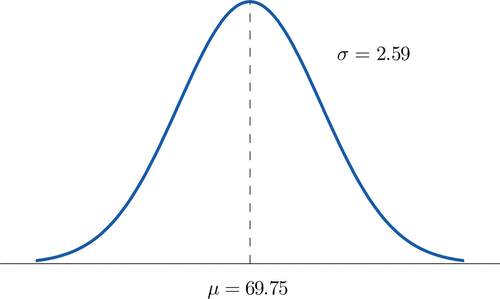# 5.1: Continuous Random Variables

Learning Objectives

• To learn the concept of the probability distribution of a continuous random variable, and how it is used to compute probabilities.
• To learn basic facts about the family of normally distributed random variables.

## The Probability Distribution of a Continuous Random Variable

For a discrete random variable $$X$$ the probability that $$X$$ assumes one of its possible values on a single trial of the experiment makes good sense. This is not the case for a continuous random variable. For example, suppose $$X$$ denotes the length of time a commuter just arriving at a bus stop has to wait for the next bus. If buses run every $$30$$ minutes without fail, then the set of possible values of $$X$$ is the interval denoted $$\left [ 0,30 \right ]$$, the set of all decimal numbers between $$0$$ and $$30$$. But although the number $$7.211916$$ is a possible value of $$X$$, there is little or no meaning to the concept of the probability that the commuter will wait precisely $$7.211916$$ minutes for the next bus. If anything the probability should be zero, since if we could meaningfully measure the waiting time to the nearest millionth of a minute it is practically inconceivable that we would ever get exactly $$7.211916$$ minutes. More meaningful questions are those of the form: What is the probability that the commuter's waiting time is less than $$10$$ minutes, or is between $$5$$ and $$10$$ minutes? In other words, with continuous random variables one is concerned not with the event that the variable assumes a single particular value, but with the event that the random variable assumes a value in a particular interval.

Definition: density function

The probability distribution of a continuous random variable $$X$$ is an assignment of probabilities to intervals of decimal numbers using a function $$f(x)$$, called a density function, in the following way: the probability that $$X$$ assumes a value in the interval $$\left [ a,b\right ]$$ is equal to the area of the region that is bounded above by the graph of the equation $$y=f(x)$$, bounded below by the x-axis, and bounded on the left and right by the vertical lines through $$a$$ and $$b$$, as illustrated in Figure $$\PageIndex{1}$$.Figure $$\PageIndex{1}$$: Probability Given as Area of a Region under a Curve

This definition can be understood as a natural outgrowth of the discussion in Section 2.1.3. There we saw that if we have in view a population (or a very large sample) and make measurements with greater and greater precision, then as the bars in the relative frequency histogram become exceedingly fine their vertical sides merge and disappear, and what is left is just the curve formed by their tops, as shown in Figure 2.1.5. Moreover the total area under the curve is $$1$$, and the proportion of the population with measurements between two numbers $$a$$ and $$b$$ is the area under the curve and between $$a$$ and $$b$$, as shown in Figure 2.1.6. If we think of $$X$$ as a measurement to infinite precision arising from the selection of any one member of the population at random, then $$P(a<X<b)$$is simply the proportion of the population with measurements between $$a$$ and $$b$$, the curve in the relative frequency histogram is the density function for $$X$$, and we arrive at the definition just above.

• Every density function $$f(x)$$ must satisfy the following two conditions:
• For all numbers $$x$$, $$f(x)\geq 0$$, so that the graph of $$y=f(x)$$ never drops below the x-axis.
• The area of the region under the graph of $$y=f(x)$$ and above the $$x$$-axis is $$1$$.

Because the area of a line segment is $$0$$, the definition of the probability distribution of a continuous random variable implies that for any particular decimal number, say $$a$$, the probability that $$X$$ assumes the exact value a is $$0$$. This property implies that whether or not the endpoints of an interval are included makes no difference concerning the probability of the interval.

For any continuous random variable $$X$$:

$P(a\leq X\leq b)=P(a<X\leq b)=P(a\leq X<b)=P(a<X<b)$

Example $$\PageIndex{1}$$

A random variable $$X$$ has the uniform distribution on the interval $$\left [ 0,1\right ]$$: the density function is $$f(x)=1$$ if $$x$$ is between $$0$$ and $$1$$ and $$f(x)=0$$ for all other values of $$x$$, as shown in Figure $$\PageIndex{2}$$.Figure $$\PageIndex{2}$$: Uniform Distribution on [0,1].
1. Find $$P(X > 0.75)$$, the probability that $$X$$ assumes a value greater than $$0.75$$.
2. Find $$P(X \leq 0.2)$$, the probability that $$X$$ assumes a value less than or equal to $$0.2$$.
3. Find $$P(0.4 < X < 0.7)$$, the probability that $$X$$ assumes a value between $$0.4$$ and $$0.7$$.

Solution:

1. $$P(X > 0.75)$$ is the area of the rectangle of height $$1$$ and base length $$1-0.75=0.25$$, hence is $$base\times height=(0.25)\cdot (1)=0.25$$. See Figure $$\PageIndex{3a}$$.
2. $$P(X \leq 0.2)$$ is the area of the rectangle of height $$1$$ and base length $$0.2-0=0.2$$, hence is $$base\times height=(0.2)\cdot (1)=0.2$$. See Figure $$\PageIndex{3b}$$.
3. $$P(0.4 < X < 0.7)$$ is the area of the rectangle of height $$1$$ and length $$0.7-0.4=0.3$$, hence is $$base\times height=(0.3)\cdot (1)=0.3$$. See Figure $$\PageIndex{3c}$$.Figure $$\PageIndex{3}$$: Probabilities from the Uniform Distribution on [0,1]

Example $$\PageIndex{2}$$

A man arrives at a bus stop at a random time (that is, with no regard for the scheduled service) to catch the next bus. Buses run every $$30$$ minutes without fail, hence the next bus will come any time during the next $$30$$ minutes with evenly distributed probability (a uniform distribution). Find the probability that a bus will come within the next $$10$$ minutes.

Solution:

The graph of the density function is a horizontal line above the interval from $$0$$ to $$30$$ and is the $$x$$-axis everywhere else. Since the total area under the curve must be $$1$$, the height of the horizontal line is $$1/30$$ (Figure $$\PageIndex{4}$$). The probability sought is $$P(0\leq X\leq 10)$$.By definition, this probability is the area of the rectangular region bounded above by the horizontal line $$f(x)=1/30$$, bounded below by the $$x$$-axis, bounded on the left by the vertical line at $$0$$ (the $$y$$-axis), and bounded on the right by the vertical line at $$10$$. This is the shaded region in Figure $$\PageIndex{4}$$. Its area is the base of the rectangle times its height, $$(10)\cdot (1/30)=1/3$$. Thus $$P(0\leq X\leq 10)=1/3$$.Figure $$\PageIndex{4}$$: Probability of Waiting At Most 10 Minutes for a Bus

## Normal Distributions

Most people have heard of the “bell curve.” It is the graph of a specific density function $$f(x)$$ that describes the behavior of continuous random variables as different as the heights of human beings, the amount of a product in a container that was filled by a high-speed packing machine, or the velocities of molecules in a gas. The formula for $$f(x)$$ contains two parameters $$\mu$$ and $$\sigma$$ that can be assigned any specific numerical values, so long as $$\sigma$$ is positive. We will not need to know the formula for $$f(x)$$, but for those who are interested it is

$f(x)=\frac{1}{\sqrt{2\pi \sigma ^2}}e^{-\frac{1}{2}(\mu -x)^2/\sigma ^2}$

where $$\pi \approx 3.14159$$ and $$e\approx 2.71828$$ is the base of the natural logarithms.

Each different choice of specific numerical values for the pair $$\mu$$ and $$\sigma$$ gives a different bell curve. The value of $$\mu$$ determines the location of the curve, as shown in Figure $$\PageIndex{5}$$. In each case the curve is symmetric about $$\mu$$.Figure $$\PageIndex{5}$$: Bell Curves with σ = 0.25 and Different Values of μ

The value of $$\sigma$$ determines whether the bell curve is tall and thin or short and squat, subject always to the condition that the total area under the curve be equal to $$1$$. This is shown in Figure $$\PageIndex{6}$$ , where we have arbitrarily chosen to center the curves at $$\mu=6$$.Figure $$\PageIndex{6}$$: Bell Curves with $$\mu =6$$ and Different Values of $$\sigma$$.

Definition: normal distribution

The probability distribution corresponding to the density function for the bell curve with parameters $$\mu$$ and $$\sigma$$ is called the normal distribution with mean $$\mu$$ and standard deviation $$\sigma$$.

Definition: normally distributed random variable

A continuous random variable whose probabilities are described by the normal distribution with mean $$\mu$$ and standard deviation $$\sigma$$ is called a normally distributed random variable, or a normal random variable for short, with mean $$\mu$$ and standard deviation $$\sigma$$.

Figure $$\PageIndex{7}$$ shows the density function that determines the normal distribution with mean $$\mu$$ and standard deviation $$\sigma$$. We repeat an important fact about this curve: The density curve for the normal distribution is symmetric about the mean.Figure $$\PageIndex{7}$$: Density Function for a Normally Distributed Random Variable with Mean $$\mu$$ and Standard Deviation $$\sigma$$

Example $$\PageIndex{3}$$

Heights of $$25$$-year-old men in a certain region have mean $$69.75$$ inches and standard deviation $$2.59$$ inches. These heights are approximately normally distributed. Thus the height $$X$$ of a randomly selected $$25$$-year-old man is a normal random variable with mean $$\mu = 69.75$$ and standard deviation $$\sigma = 2.59$$. Sketch a qualitatively accurate graph of the density function for $$X$$. Find the probability that a randomly selected $$25$$-year-old man is more than $$69.75$$ inches tall.

Solution:

The distribution of heights looks like the bell curve in Figure $$\PageIndex{8}$$. The important point is that it is centered at its mean, $$69.75$$, and is symmetric about the mean.Figure $$\PageIndex{8}$$: Density Function for Heights of $$25$$-Year-Old Men

Since the total area under the curve is $$1$$, by symmetry the area to the right of $$69.75$$ is half the total, or $$0.5$$. But this area is precisely the probability $$P(X > 69.75)$$, the probability that a randomly selected $$25$$-year-old man is more than $$69.75$$ inches tall. We will learn how to compute other probabilities in the next two sections.

## Key Takeaway

• For a continuous random variable $$X$$ the only probabilities that are computed are those of $$X$$ taking a value in a specified interval.
• The probability that $$X$$ take a value in a particular interval is the same whether or not the endpoints of the interval are included.
• The probability $$P(a<X<b)$$, that $$X$$ take a value in the interval from $$a$$ to $$b$$, is the area of the region between the vertical lines through $$a$$ and $$b$$, above the $$x$$-axis, and below the graph of a function $$f(x)$$ called the density function.
• A normally distributed random variable is one whose density function is a bell curve.
• Every bell curve is symmetric about its mean and lies everywhere above the $$x$$-axis, which it approaches asymptotically (arbitrarily closely without touching).